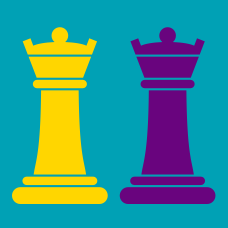Logic

# Chess Tactical: Level 3 Challenges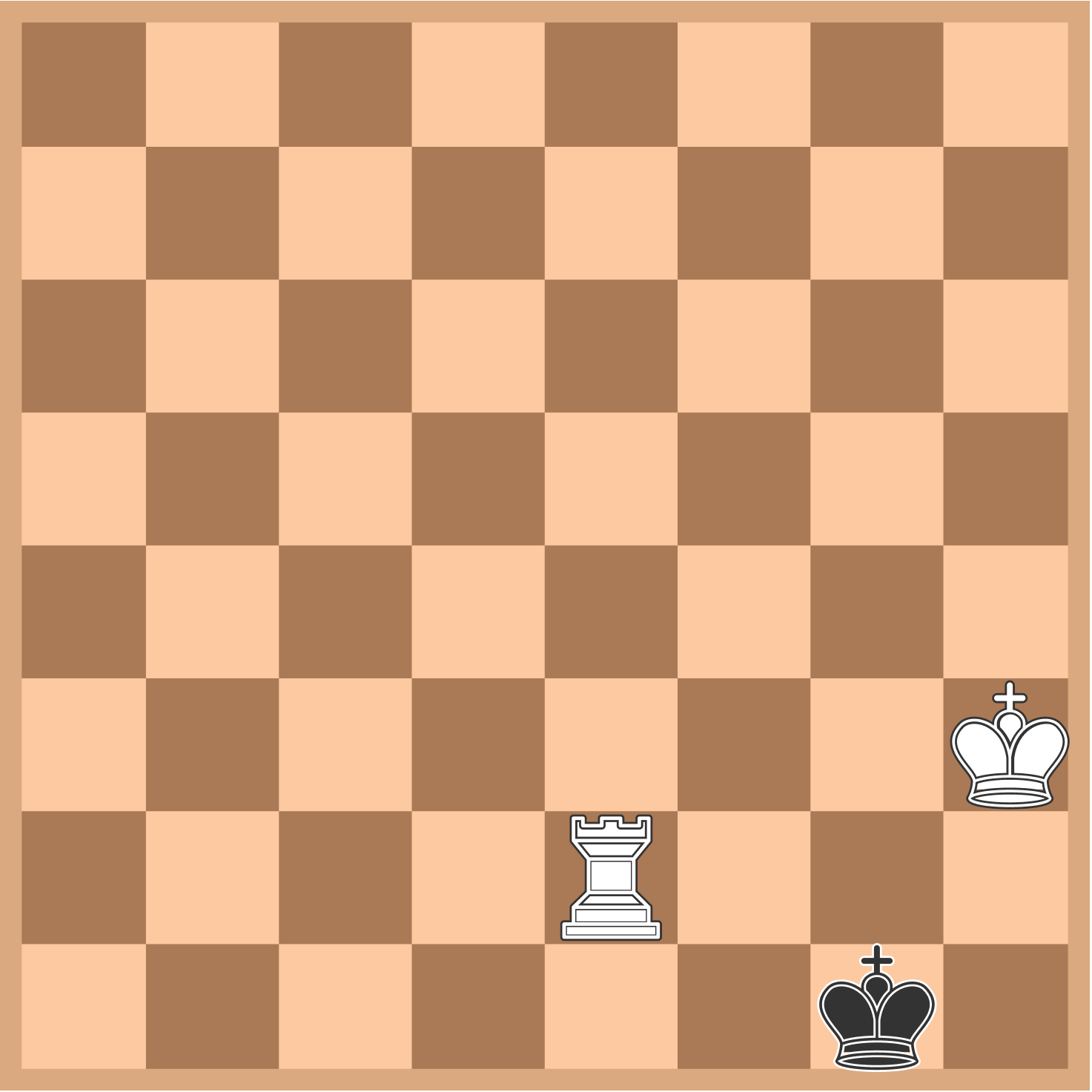Find the minimum number of moves for White to checkmate Black.

White starts, and Black plays optimally.

In the position below, how can White give checkmate in two moves?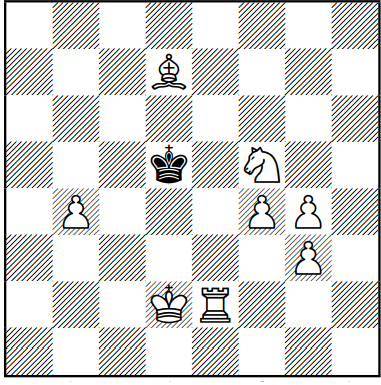The target square of the first move can be represented as a coordinate $$(x,y)$$, where the bottom left corner square is at $$(1,1)$$. Input the product of $$x$$ and $$y$$ as your answer.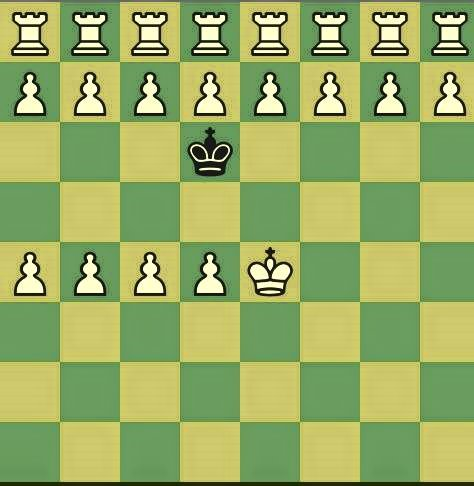It's White turn to move, find the minimum number of moves for White to move to win the game.

Note that both players played optimally and that White moves up (pawn).

Assume for the sake of this puzzle that there are more white pieces than originally intended.

###### Designed by Elangraka Elang.

It is White's move. What must White play in order to mate in 3?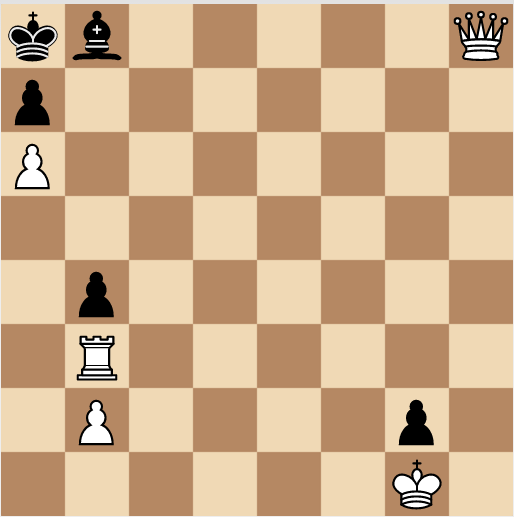The bottom left corner square has coordinates $$(1, 1)$$. Each piece has a value: $$\text{Queen}=10$$, $$\text{Rook}=5$$, $$\text{Pawn}=1$$ and $$\text{King}=4$$.

Submit your answer as $$V\times { x }_{ 1 }\times { y }_{ 1 }$$, where $$V$$ denotes the value of the piece that White must move and the coordinates of the square where it has to go are $$\left( { x }_{ 1 },{ y }_{ 1 } \right)$$.

Clarification: Black plays optimally.

###### This is the second problem of the set Mate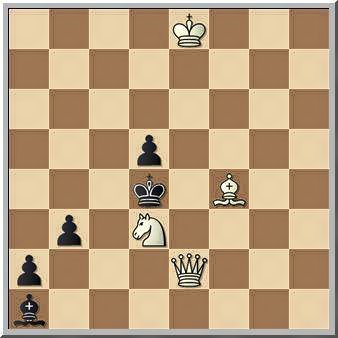It's White turn, what is the minimum number of moves White should make to checkmate

Assume that both players played optimally and that Black moves down (pawn).

###### Designed by Bastian Ismail.
×

Problem Loading...

Note Loading...

Set Loading...